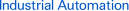Page top# time constant

## Definition

The appropriate product category
First-order delay elements vary with the characteristics of an exponential function. A simple way to express the speed of change is thus desirable. A way called the “time constant” is used. The time constant estimates the time required to reach 100% if the slop at startup (0%) is maintained. It is, however, difficult to find the time constant from the startup slop. If the value after a time equivalent to the time constant in measured in first-order delay calculation, it will be approximately 63%. Thus, the time required to reach 63% is measured and used to calculate the time constant.
The appropriate product category
In a linear primary delay system, the time required for an output to reach approximately 63% of the total change when a step-wise input is applied.
The appropriate product category
Time required to reach approximately 63% of the final value when a step input is entered in a first-order linear delay system.